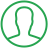# How To Generate Random String In Java

Last Updated : Jul 1, 2023In this article we will show you the solution of how to generate random string in java, the pseudo-random double values are generated using the Java Math.random() function and range from 0.0 to 1.0.

However, you may combine this strategy with additional strategies to produce a random string.

The index of the characters in a predetermined character pool can be represented by an integer by converting the random double value.

After that, you can join these characters to form a random string of any length.

You may use Math.random() to create a string that is random in Java by repeating this procedure.

The strings generated by Math.random() should not be used in applications that require security because they are not truly random, it is important to keep in mind.

## Step By Step Guide On How To Generate Random String In Java :-

```public class RandomString {
private static final String ALPHA_NUMERIC_STRING = "ABCDEFGHIJKLMNOPQRSTUVWXYZ0123456789abcdefghijklmnopqrstuvxyz";
public static String getAlphaNumericString(int n) {
StringBuilder sb = new StringBuilder(n);
for (int i = 0; i < n; i++) {
int index = (int)(ALPHA_NUMERIC_STRING.length() * Math.random());
sb.append(ALPHA_NUMERIC_STRING.charAt(index));
}
return sb.toString();
}
public static void main(String[] args) {
int n = 20;
System.out.println(RandomString.getAlphaNumericString(n));
}
}```
1. You can see that we write java code to generate a random string using the Math.random() function.
2. Next, we create a class with public access level called RandomString.
3. Then, we define the ALPHA_NUMERIC_STRING private static final String variable, which is a collection of all the characters (letters and digits) that can be used to create random strings.
4. The next step is to create a public static method called getAlphaNumericString that accepts an integer n as an input and returns a random string made up of characters from ALPHA_NUMERIC_STRING.
5. A StringBuilder object with a capacity of n is created inside the method and given the name sb. This object will be used to construct the random string.
6. Next, a for loop is used to generate a random index between 0 and ALPHA_NUMERIC_STRING for each iteration.Use Math.random() to generate length()-1, then multiply the result by the length of ALPHA_NUMERIC_STRING.
7. Next, use charAt() to retrieve the character at that index from ALPHA_NUMERIC_STRING after converting the random index to an int.
8. The character is then added to sb using the append() function.
9. Use toString() to convert sb to a string after the loop and then return the resultant random string.
10. The program's entry point is then defined as a public static main method.
11. Then, to determine the preferred length of the random string, we create an integer variable n having a value of 20.
12. Then, to create a random string of length n, call the getAlphaNumericString() function of the RandomString class while supplying n as an argument.
13. The last print uses System.out.println() to output the created random string to the console.

## Conclusion :-

As a result, we have successfully learnt the Java notion of creating random strings.

Additionally, we learnt how to use Math.random() to create a random string of alphanumeric characters.

However, due to the generated strings' lack of true randomness and potential suitability for applications requiring security, care should be taken.

Use methods like java.security.SecureRandom, which are more secure. The resulting string's length and structure can also be modified to meet specific needs.

Although the code provides a straightforward method for producing random strings, further considerations may be required depending on the use cases and security requirements.

I hope this article on how to generate random string in java helps you and the steps and method mentioned above are easy to follow and implement.

##About Riya

A recent graduate with a Bachelor of Technology (B.Tech) in Computer Science from India. She is passionate about leveraging technology to solve real-world problems. With a strong foundation and experience in programming languages such as Python, Django, HTML, CSS, and JavaScript, java, php and have honed her skills through hands-on projects and coursework.